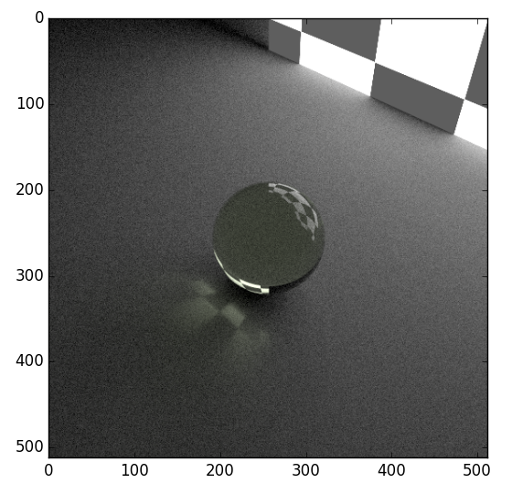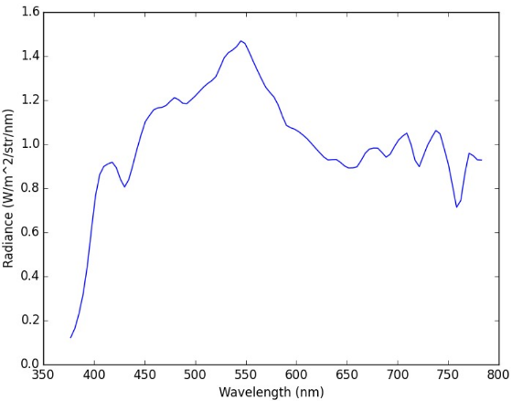# 5. Quickstart Guide¶

This example is based on the demo file demos/quickstart/demo_lambert.py. It outlines the typical workflow used in raysect.

## 5.1. Create Primitives¶

Set-up your primitives by defining materials, meshes, etc:

# Box defining the ground plane
ground = Box(lower=Point3D(-50, -1.51, -50), upper=Point3D(50, -1.5, 50), material=Lambert())

# checker board wall that acts as emitter
emitter = Box(lower=Point3D(-10, -10, 10), upper=Point3D(10, 10, 10.1),
material=Checkerboard(4, d65_white, d65_white, 0.1, 2.0), transform=rotate(45, 0, 0))

# Sphere
# Note that the sphere must be displaced slightly above the ground plane to prevent numerically issues that could
# cause a light leak at the intersection between the sphere and the ground.
sphere = Sphere(radius=1.5, transform=translate(0, 0.0001, 0), material=schott("N-BK7"))


Add an observer and configure its sampling settings. All of these camera settings have sensible defaults, The camera settings will be explained in detail in another section:

# processing pipeline (human vision like camera response)
rgb = RGBPipeline2D()

# camera
camera = PinholeCamera(pixels=(512, 512), fov=45, pipeline=[rgb], transform=translate(0, 10, -10) * rotate(0, -45, 0))

# camera - pixel sampling settings
camera.pixel_samples = 250
camera.min_wavelength = 375.0
camera.max_wavelength = 740.0
camera.spectral_bins = 15
camera.spectral_rays = 1


## 5.3. Build Scenegraph¶

Assemble the scene-graph by linking primitives and observers to the World. Set their transforms:

world = World()

sphere.parent = world
ground.parent = world
emitter.parent = world
camera.parent = world


## 5.4. Observe()¶

Call observe() on an Observer or trace a ray manually:

plt.ion()
camera.observe()

plt.ioff()
rgb.save("render.png")
rgb.display()


The resulting image should render like this.## 5.5. Simulated Spectrum¶

Lets simulate measuring a spectrum by launching a single ray:

ray = Ray(origin=Point3D(0, 0, -5),
direction=Vector3D(0, 0, 1),
min_wavelength=375,
max_wavelength=785,
bins=100)

ray.trace(world)


The resulting plot should look something like this.Due to the statistical nature of the path tracer, you may need to run the trace command more than once until you find a path that intersects with the light source.

You can ask the ray to trace repeatedly using the sample method instead. This will combine the results of multiple paths:

ray.sample(world, 10000)